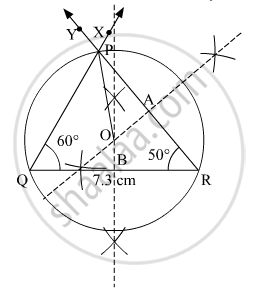Advertisement Remove all ads

# Construct δ Pqr Such that ∠ P = 70 ∘ , ∠ R = 50 ∘ , Qr = 7.3 Cm and Constructs Its Circumcircle. - Geometry

Diagram

Construct Δ PQR such that ∠P = 70° , ∠R = 50° , QR = 7.3 cm  and constructs its circumcircle.

Advertisement Remove all ads

#### Solution

By angle sum property of triangles,

In Δ PQR,

∠P + ∠Q +∠R = 180°

⇒ 70° + ∠Q + 50° = 180°

⇒ ∠Q = 60°

Steps of construction:
1. Draw a line QR = 7.3 cm.
2. With Q as centre, draw an angle of 60° using the protractor. Similarly, draw an angle of 50° with R as centre.
Where the rays RY and XQ meet, name the point as P.
Thus, Δ PQR is obtained.
3. Draw the perpendicular bisectors of the lines PR and QR. Let these perpendicular bisectors meet at point O.
4. With O as centre and OP as radius, construct a circle touching all the vertices of the Δ PQR.
This circle is thus the required circumcircle.Is there an error in this question or solution?
Advertisement Remove all ads

#### APPEARS IN

Balbharati Mathematics 2 Geometry 9th Standard Maharashtra State Board
Chapter 6 Circle
Practice Set 6.3 | Q 2 | Page 86
Advertisement Remove all ads
Advertisement Remove all ads
Share
Notifications

View all notifications

Forgot password?